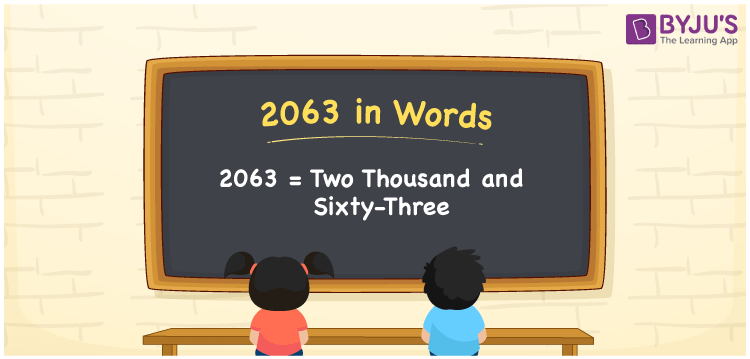# 2063 in words

2063 in words is written as Two Thousand and Sixty Three. 2063 represents the count or value. The article on Counting Numbers can give you an idea about count or counting. The number 2063 is used in expressions that relate to money, distance, length, year and others. Let us consider an example for 2063. ”Grocery shopping came up to Two Thousand and Sixty Three rupees.”

 2063 in words Two Thousand and Sixty Three Two Thousand and Sixty Three in Numbers 2063

## 2063 in English Words## How to Write 2063 in Words?

We can convert 2063 to words using a place value chart. The number 2063 has 4 digits, so let’s make a chart that shows the place value up to 4 digits.

 Thousands Hundreds Tens Ones 2 0 6 3

Thus, we can write the expanded form as:

2 × Thousand + 0 × Hundred + 6 × Ten + 3 × One

= 2 × 1000 + 0 × 100 + 6 × 10 + 3 × 1

= 2063

= Two Thousand and Sixty Three.

2063 is the natural number that is succeeded by 2062 and preceded by 2064.

2063 in words – Two Thousand and Sixty Three.

Is 2063 an odd number? – Yes.

Is 2063 an even number? – No.

Is 2063 a perfect square number? – No.

Is 2063 a perfect cube number? – No.

Is 2063 a prime number? – Yes.

Is 2063 a composite number? – No.

## Solved Example

1. Write the number 2063 in expanded form

Solution: 2 × 1000 + 0 × 100 + 6 × 10 + 3 × 1

We can write 2063 = 2000 + 000 + 60 + 3

= 2 × 1000 + 0 × 100 + 6 × 10 + 3 × 1

## Frequently Asked Questions on 2063 in words

Q1

### How to write 2063 in words?

2063 in words is written as Two Thousand and Sixty Three.
Q2

### Is 2063 a perfect square number?

No. 2063 is not a perfect square number.
Q3

### Is 2063 a prime number?

Yes. 2063 is a prime number.